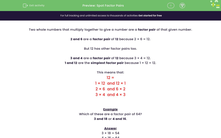# Recognise Factor Pairs

In this worksheet, students will identify the factor pairs for the given number.Key stage:  KS 2

Curriculum topic:   Number: Multiplication and Division

Curriculum subtopic:   Recognise/Use Pairs in Mental Calculation

Difficulty level:#### Worksheet Overview

Two whole numbers that multiply together to give a number are a factor pair of that given number.

2 and 6 are a factor pair of 12 because 2 × 6 = 12.

But 12 has other factor pairs too.

3 and 4 are a factor pair of 12 because 3 × 4 = 12.

1 and 12 are the simplest factor pair because 1 × 12 = 12.

This means that:

12 =

1 × 12  or 12 × 1

2 × 6  or 6 × 2

3 × 4  or 4 × 3

Example

Which of these is a factor pair of 64?

3 and 18 or 4 and 16.

3 × 18 = 54

4 × 16 = 64

So, 4 and 16 are a factor pair of 64.

All the other factor pairs of 64 are:

1 x 64 or 64 x 1

2 x 32 or 32 x 2

8 x 8

Let's have a go at spotting factor pairs in the questions that follow.### What is EdPlace?

We're your National Curriculum aligned online education content provider helping each child succeed in English, maths and science from year 1 to GCSE. With an EdPlace account you’ll be able to track and measure progress, helping each child achieve their best. We build confidence and attainment by personalising each child’s learning at a level that suits them.

Get started